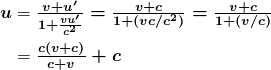Chapter 13 Special Relativity

# 13.4 Relativistic Addition of Velocities

### Summary

• Calculate relativistic Doppler shift.

If you’ve ever seen a kayak move down a fast-moving river, you know that remaining in the same place would be hard. The river current pulls the kayak along. Pushing the oars back against the water can move the kayak forward in the water, but that only accounts for part of the velocity. The kayak’s motion is an example of classical addition of velocities. In classical physics, velocities add as vectors. The kayak’s velocity is the vector sum of its velocity relative to the water and the water’s velocity relative to the riverbank.

For simplicity, we restrict our consideration of velocity addition to one-dimensional motion. Classically, velocities add like regular numbers in one-dimensional motion. (See Figure 2.) Suppose, for example, a girl is riding in a sled at a speed 1.0 m/s relative to an observer. She throws a snowball first forward, then backward at a speed of 1.5 m/s relative to the sled. We denote direction with plus and minus signs in one dimension; in this example, forward is positive. Let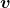be the velocity of the sled relative to the Earth,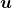the velocity of the snowball relative to the Earth-bound observer, and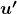the velocity of the snowball relative to the sled.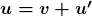Thus, when the girl throws the snowball forward,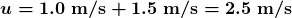. It makes good intuitive sense that the snowball will head towards the Earth-bound observer faster, because it is thrown forward from a moving vehicle. When the girl throws the snowball backward,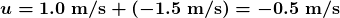. The minus sign means the snowball moves away from the Earth-bound observer.

The second postulate of relativity (verified by extensive experimental observation) says that classical velocity addition does not apply to light. Imagine a car traveling at night along a straight road, as in Figure 3. If classical velocity addition applied to light, then the light from the car’s headlights would approach the observer on the sidewalk at a speed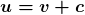. But we know that light will move away from the car at speed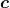relative to the driver of the car, and light will move towards the observer on the sidewalk at speed, too.

Either light is an exception, or the classical velocity addition formula only works at low velocities. The latter is the case. The correct formula for one-dimensional relativistic velocity addition is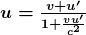where v is the relative velocity between two observers, u is the velocity of an object relative to one observer, and u’ is the velocity relative to the other observer. (For ease of visualization, we often choose to measure u in our reference frame, while someone moving at v relative to us measures u’.) Note that the term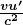becomes very small at low velocities, andgives a result very close to classical velocity addition. As before, we see that classical velocity addition is an excellent approximation to the correct relativistic formula for small velocities. No wonder that it seems correct in our experience.

### Example 1: Showing that the Speed of Light towards an Observer is Constant (in a Vacuum): The Speed of Light is the Speed of Light

Suppose a spaceship heading directly towards the Earth at half the speed of light sends a signal to us on a laser-produced beam of light. Given that the light leaves the ship at speedas observed from the ship, calculate the speed at which it approaches the Earth.

Strategy

Because the light and the spaceship are moving at relativistic speeds, we cannot use simple velocity addition. Instead, we can determine the speed at which the light approaches the Earth using relativistic velocity addition.

Solution

1. Identify the knowns.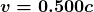;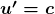2. Identify the unknown.3. Choose the appropriate equation.4. Plug the knowns into the equation.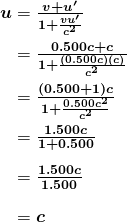Discussion

Relativistic velocity addition gives the correct result. Light leaves the ship at speedand approaches the Earth at speed. The speed of light is independent of the relative motion of source and observer, whether the observer is on the ship or Earth-bound.

Velocities cannot add to greater than the speed of light, provided thatis less thananddoes not exceed. The following example illustrates that relativistic velocity addition is not as symmetric as classical velocity addition.

### Example 2: Comparing the Speed of Light towards and away from an Observer: Relativistic Package Delivery

Suppose the spaceship in the previous example is approaching the Earth at half the speed of light and shoots a canister at a speed of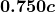. (a) At what velocity will an Earth-bound observer see the canister if it is shot directly towards the Earth? (b) If it is shot directly away from the Earth? (See Figure 5.)

Strategy

Because the canister and the spaceship are moving at relativistic speeds, we must determine the speed of the canister by an Earth-bound observer using relativistic velocity addition instead of simple velocity addition.

Solution for (a)

1. Identify the knowns.;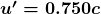2. Identify the unknown.3. Choose the appropriate equation.4. Plug the knowns into the equation.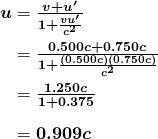Solution for (b)

1. Identify the knowns.;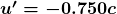2. Identify the unknown.3. Choose the appropriate equation.4. Plug the knowns into the equation.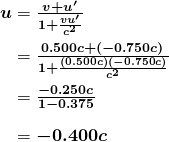Discussion

The minus sign indicates velocity away from the Earth (in the opposite direction from), which means the canister is heading towards the Earth in part (a) and away in part (b), as expected. But relativistic velocities do not add as simply as they do classically. In part (a), the canister does approach the Earth faster, but not at the simple sum of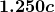. The total velocity is less than you would get classically. And in part (b), the canister moves away from the Earth at a velocity of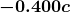, which is faster than the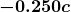you would expect classically. The velocities are not even symmetric. In part (a) the canister moves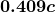faster than the ship relative to the Earth, whereas in part (b) it moves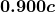slower than the ship.

# Doppler Shift

Although the speed of light does not change with relative velocity, the frequencies and wavelengths of light do. First discussed for sound waves, a Doppler shift occurs in any wave when there is relative motion between source and observer.

### Relativistic Doppler Effects

The observed wavelength of electromagnetic radiation is longer (called a red shift) than that emitted by the source when the source moves away from the observer and shorter (called a blue shift) when the source moves towards the observer.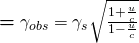.

In the Doppler equation,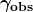is the observed wavelength,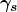is the source wavelength, andis the relative velocity of the source to the observer. The velocity u is positive for motion away from an observer and negative for motion toward an observer. In terms of source frequency and observed frequency, this equation can be written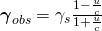Notice that the – and + signs are different than in the wavelength equation.

### Career Connection: Astronomer

If you are interested in a career that requires a knowledge of special relativity, there’s probably no better connection than astronomy. Astronomers must take into account relativistic effects when they calculate distances, times, and speeds of black holes, galaxies, quasars, and all other astronomical objects. To have a career in astronomy, you need at least an undergraduate degree in either physics or astronomy, but a Master’s or doctoral degree is often required. You also need a good background in high-level mathematics.

### Example 3: Calculating a Doppler Shift: Radio Waves from a Receding Galaxy

Suppose a galaxy is moving away from the Earth at a speed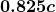. It emits radio waves with a wavelength of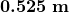. What wavelength would we detect on the Earth?

Strategy

Because the galaxy is moving at a relativistic speed, we must determine the Doppler shift of the radio waves using the relativistic Doppler shift instead of the classical Doppler shift.

Solution

1. Identify the knowns.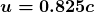;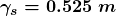2. Identify the unknown.3. Choose the appropriate equation.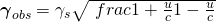4. Plug the knowns into the equation.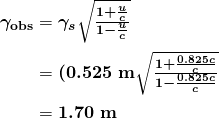Discussion

Because the galaxy is moving away from the Earth, we expect the wavelengths of radiation it emits to be redshifted. The wavelength we calculated is 1.70 m, which is redshifted from the original wavelength of 0.525 m.

The relativistic Doppler shift is easy to observe. This equation has everyday applications ranging from Doppler-shifted radar velocity measurements of transportation to Doppler-radar storm monitoring. In astronomical observations, the relativistic Doppler shift provides velocity information such as the motion and distance of stars.

1: Suppose a space probe moves away from the Earth at a speed 0.350c . It sends a radio wave message back to the Earth at a frequency of 1.50 GHz. At what frequency is the message received on the Earth?

# Section Summary

• With classical velocity addition, velocities add like regular numbers in one-dimensional motion: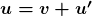, whereis the velocity between two observers,is the velocity of an object relative to one observer, andis the velocity relative to the other observer.
• Velocities cannot add to be greater than the speed of light. Relativistic velocity addition describes the velocities of an object moving at a relativistic speed: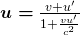### Conceptual Questions

1: Explain the meaning of the terms “red shift” and “blue shift” as they relate to the relativistic Doppler effect.

2: What happens to the relativistic Doppler effect when relative velocity is zero? Is this the expected result?

3: Is the relativistic Doppler effect consistent with the classical Doppler effect in the respect thatis larger for motion away?

4: All galaxies farther away than about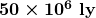exhibit a red shift in their emitted light that is proportional to distance, with those farther and farther away having progressively greater red shifts. What does this imply, assuming that the only source of red shift is relative motion? (Hint: At these large distances, it is space itself that is expanding, but the effect on light is the same.)

### Problems & Exercises

1: Suppose a spaceship heading straight towards the Earth atcan shoot a canister atrelative to the ship. (a) What is the velocity of the canister relative to the Earth, if it is shot directly at the Earth? (b) If it is shot directly away from the Earth?

2: Repeat the previous problem with the ship heading directly away from the Earth.

3: If a spaceship is approaching the Earth at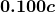and a message capsule is sent toward it atrelative to the Earth, what is the speed of the capsule relative to the ship?

4: (a) Suppose the speed of light were only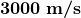. A jet fighter moving toward a target on the ground at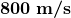shoots bullets, each having a muzzle velocity of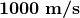. What are the bullets’ velocity relative to the target? (b) If the speed of light was this small, would you observe relativistic effects in everyday life? Discuss.

5: If a galaxy moving away from the Earth has a speed of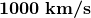and emits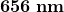light characteristic of hydrogen (the most common element in the universe). (a) What wavelength would we observe on the Earth? (b) What type of electromagnetic radiation is this? (c) Why is the speed of the Earth in its orbit negligible here?

6: A space probe speeding towards the nearest star moves atand sends radio information at a broadcast frequency of 1.00 GHz. What frequency is received on the Earth?

7: If two spaceships are heading directly towards each other at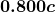, at what speed must a canister be shot from the first ship to approach the other at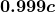as seen by the second ship?

8: Two planets are on a collision course, heading directly towards each other at. A spaceship sent from one planet approaches the second atas seen by the second planet. What is the velocity of the ship relative to the first planet?

9: When a missile is shot from one spaceship towards another, it leaves the first at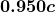and approaches the other at. What is the relative velocity of the two ships?

10: What is the relative velocity of two spaceships if one fires a missile at the other atand the other observes it to approach at?

11: Near the center of our galaxy, hydrogen gas is moving directly away from us in its orbit about a black hole. We receive 1900 nm electromagnetic radiation and know that it was 1875 nm when emitted by the hydrogen gas. What is the speed of the gas?

12: A highway patrol officer uses a device that measures the speed of vehicles by bouncing radar off them and measuring the Doppler shift. The outgoing radar has a frequency of 100 GHz and the returning echo has a frequency 15.0 kHz higher. What is the velocity of the vehicle? Note that there are two Doppler shifts in echoes. Be certain not to round off until the end of the problem, because the effect is small.

13: Prove that for any relative velocitybetween two observers, a beam of light sent from one to the other will approach at speed(provided thatis less than, of course).

## Glossary

the method of adding velocities when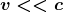; velocities add like regular numbers in one-dimensional motion: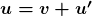, whereis the velocity between two observers, uu is the velocity of an object relative to one observer, andis the velocity relative to the other observer
the method of adding velocities of an object moving at a relativistic speed:, whereis the relative velocity between two observers,is the velocity of an object relative to one observer, andis the velocity relative to the other observer.

### Solutions

1:  1.04  GHz

Problems & Exercises

1: (a) 0.909c (b) 0.400c

3: 0.198c

5: a) 658 nm  b) red c)  v/c = 9.92×10-5  (negligible)

7: 0.991 c

9: -0.696c   0.01324c

13: u’=c , so